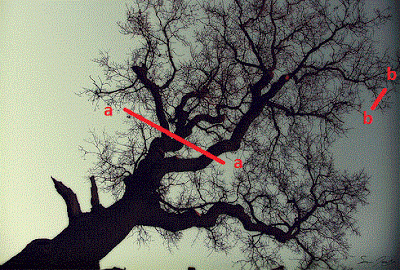## Posts

Showing posts from March, 2011

### Uniformitarianism and the FractalUniformitarianism : The key to the past can be found in the present. I have a strong interest in geography and geology and it was here where I first read of Hutton’s uniformity, I soon found – after teaching development economics - that this principle may be more universal, and may show in economics too. The law of uniformitarianism reveals itself in the fractal. To describe a fractal, one would eventually cover the principle, only instead of reading as above - the key the past can be found in the present - it would read as the key to the present (scale) can be found in the small scale – or conversely the large scale, assuming. ceteris paribus approach, (holding all else constant or frozen). In the below tree fractal, the new (present) cross-section line b-b will share the same, (but different) as the old (past) cross-section line a-a. Scale is the only difference - both in time (age) and size. Fractal Demonstration of Uniformity It is another insight from the fundamental ch

### Macro and Micro and the FractalUpdated 29th Nov 2012 There are many insights - in relation to macro/micro - that can be taken from the fractal . Firstly, and importantly, when viewing a fractal (in isolation) scale cannot be discerned, the object maybe any size at all, from infinity small to infinitly large; the object shares the fractal charactoristic of being 'same' but 'different' at every scale. What can be discerned is the shape of the object, and from this an attempt can be made to deduce an understanding of the process to produce it. The object is the 'same', the examples infinitly 'different'. Examples: income, wealth, trade, selection, reproduction, specialisation and so on. We see the same but different - at all scales. These principles repeat throughout the universe and are central too to biology, and maybe even chemistry and physics also. This observation sheds light on whether there is a distinction between micro and macro : the fractal shows us that there maybe n

### Fractal Long Run Short RunThe fractal demonstrates the economic Short Run and the Long Run In line with the classical economic view of short run and the long run - best demonstrated by images of  cost curves - the fractal is the math of the said phenomena. Short Run: The development and growth of the fractal – from iteration 1 to the equilibrium iteration is the fractal (economic) Short Run. The Short Run is the effect of the starting rule, e.g. branching, or adding of triangles. The Long Run: The Long Run is the end state, the total superpostition, where all the infinite possibilities are shown : the state where equilibrium iteration shape is set – to the fully developed tree, or snowflake.